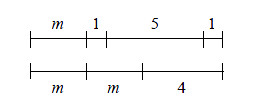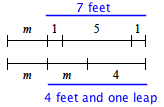### Home > MC1 > Chapter 3 > Lesson 3.4.5 > Problem3-148

3-148.

The diagram below represents an acrobat's sequence on a tightrope, where $m$ represents the distance in feet that she covers each time she does a leap.1. How long is each of her leaps? How can you tell?

Try matching up sections of the sequences to more easily determine the length of each leap.
On one tightrope she travels $7$ feet. On the other, she goes $4$ feet and one leap.
Can you find the length of the leap now?2. How long is the tightrope?

Choosing either one of the given sequences, add the lengths of all sections, including the length of the leap you just found.

$10$ feet

3. Write expressions to represent each of her two sequences across the tightrope.

To get an answer for part (b), you probably added each portion of one of the sequences together. Essentially, you were solving for the value of an expression for that sequence. Now, you just need to write that expression.

Caroline wrote the following expression for the first sequence: $m + 7$.